CiteScore 3.0
More on impact ›

# Frontiers in Mechanical Engineering## ORIGINAL RESEARCH article

Front. Mech. Eng., 17 September 2021 | https://doi.org/10.3389/fmech.2021.703019

# Acoustic Wave Reflection Control Based on Broadband Differential Phase ShiftersZifu Qin,Xiaohan Liu and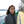Chu Ma*
• Acoustic Sensing and Functional Materials Lab, Department of Electrical and Computer Engineering, University of Wisconsin-Madison, Madison, WI, United States

Controlling the flow of acoustic waves has broad applications in acoustic imaging, communication, energy harvesting, audio systems, etc. Metasurfaces have been developed for wave control. In this work we propose the design of broadband differential phase shifters for acoustic reflected waves, which can achieve nearly constant phase shift values over a broad frequency ranges. We further demonstrate the design of a broadband differential π phase shifter that works in the frequency range of (10 kHz, 16 KHz) and its applications in acoustic metasurfaces for steering and focusing of reflected acoustic waves. The metasurfaces we designed have the following advantages: 1) The metasurface is formed with binary patterns instead of more complex phase discretization steps, thus the metasurface realization is less complex for fabrication and assembly. 2) The phase shift values of the two basic phase shifter elements are broadband, thus we do not need to fabricate new elements for each frequency. Rearranging the same elements into different location patterns would allow us to tune the working frequency of the metasurfaces.

## 1 Introduction

Controlling the flow of waves and guiding them to directions and locations of interest has long been an important area of research. Potential applications include imaging, communication, energy harvesting, audio systems, etc. Among the solutions for wave controlling problems, metamaterials demonstrate promising performance by achieving effective material properties that does not exist in nature with engineered subwavelength resonating structures in both electromagnetics Zheludev and Kivshar (2012) and acoustics (Cummer et al., 2016; Ma and Sheng, 2016). As the 2D realizations of metamaterials, metasurfaces utilize the Huygens principle to discretize the surface into subwavelength unit cells, each having designed phase and/or amplitude responses (Yu et al., 2011; Sun et al., 2012; Kildishev et al., 2013; Chen et al., 2016; Glybovski et al., 2016). In acoustics, most commonly explored metasurfaces are phase gradient metasurfaces (Li et al., 2014; Tang et al., 2014; Xie et al., 2014; Zhu et al., 2016; Li et al., 2018; Zhu et al., 2018). They are composed of phase shifters that can generate discrete values of phase shifts in the range of (0, 2π). Those phase shifters are arranged in the metasurface to form the designed phase gradient for wavefront manipulation (Zhao et al., 2013; Li et al., 2015; Wang et al., 2016), acoustic absorption (Mei et al., 2012; Ma et al., 2014; Li et al., 2016), cloaking (Zhang et al., 2013; Esfahlani et al., 2016), hologram (Xie et al., 2016), etc.

There is a common property in the acoustic phase shifters that are previously designed: the phase shift values provided by the phase shifters are frequency dependent. For example, in the work Xie et al. (2014) and Liang and Li (2012), the phase shifters are formed by curved waveguide channels that generate phase delays proportional to the total wave path lengths. For a phase shifter with a fixed length, the time delay after passing through the waveguide channel is a constant as the frequency changes, which means the phase shift is a function of frequency. Most of the previously designed phase shifters belong to this type. In this paper, we will introduce a new type of phase shifters for acoustic reflected waves in which the phase shift values do not change with frequency. Our work is inspired by the coupled transmission line design in electrical circuits (Quirarte and Starski, 1993; Guo et al., 2006; Zhang et al., 2011). Here we extend the idea from electrical circuits into acoustics with the help of acoustic transmission line theory (Beranek, 1954; Zhang et al., 2009). The designed phase shifters are then applied to metasurfaces for manipulation of acoustic reflected waves.

In the previous acoustic metasurface designs, the resolution of spatial discretization is usually larger than five unit cells per period in order to form smooth phase gradient. Such dense arrangement of unit cells limits the lateral resolution of the phase gradient. The complexity in design and fabrication of phase shifters also increases with the increasing resolution of phase response discretization. Here we propose a new way to achieve acoustic wave control using only two discrete phase shift values, 0 and π.

The rest of the paper is organized as the following: Section 2 describes the theoretical design and simulation of a broadband differential phase shifter with π phase difference between its two components. Section 3 describes the design of acoustic reflected wave metasurfaces for wave steering and focusing based on the broadband differential phase shifter designed in section 2. Section 4 summarizes the results and provide further discussion about the advantages and applications of the phase shifter and metasurface designs.

## 2 Broadband Differential π Phase Shifter for Reflected Acoustic Wave

First of all, we will demonstrate the design of a broadband differential phase shifter that generates π phase difference between its two components. Those two components will be the key elements for the binary metasurfaces we will introduce later in this work.

In Zhang et al. (2011), the authors presented the design of a Schiffman differential phase shifter for microwave circuits, which consists of two components. The output phase of each individual component is frequency-dependent. However, the output phase difference between the two components is nearly constant over a broadband frequency range. Similar as in Zhang et al. (2011), we consider a differential phase shifter for reflected acoustic wave, in which the reflected wave phase difference between two components is constant over a broadband frequency range. Here, one component is a straight acoustic waveguide with width d and length l0 (Figure 1A), and the other component is a straight acoustic waveguide (width d and length l1) with two identical Helmholtz Resonator (HR) side branches (neck r1, h1 and cavity r2, h2) (Figure 1B). We call the first component “tube” and the other component “resonator”.

FIGURE 1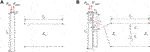FIGURE 1. System schematics and the transmission line circuit model for the two components of the phase shifter. (A) A tube and its effective circuit model as a delay line with impedance Zc. (B) A resonator and the effective circuit model at the location of the Helmholtz Resonator side branch.

The effective circuit model for the tube is a delay line of length 2l0, as shown in Figure 1A. The factor “2” is due to the fact that wave is reflected at the bottom of the tube and the total travel path length is twice the length of the tube. Assume Pin is the incident acoustic pressure, and $Poutejφ0$ is the reflected acoustic pressure. We have:

$φ0=−2kl0.(1)$

The delay line generates a phase shift −2kl0, where k = 2πf/c is the wavenumber in air.

As shown in Figure 1B, the effective circuit model for the resonator is a delay line with length 2l1 that generates phase shift value of −2kl1, and four side branches that are identical. Each side branch is composed of a capacitor and an inductor. The characteristic impedance of a tube with width d is $Zc=cρd$, where ρ is the density of the material in the tube and c is the sound velocity inside the tube. The impedance of the side branch is $Zs=1jωC+jωL$. Here, $C=r2h2ρc2$ is the effective capacitance of the cavity in the HR and $L=ρheqr1$ is the effective inductance of the neck of the HR, where heq is the modified neck length that is determined by the real neck length h1 and the end loading. We will determine heq according to simulation of the resonator. The effective acoustic impedance at the location of side branch can be written as the parallel of Zc and Zs, which is

$Z=1jωC+jωLZcZc+1jωC+jωL.(2)$

For the resonator component, We also assume Pin as the incident pressure, $Pout′ejφ1$ as the reflected pressure field. We have:

$φ1=−2kl1+4∠2ZZc+Z.(3)$

Here $2ZZc+Z$ is the transmission coefficient T at the location of the HR when the wave passes by one of the HR side branches.

The differential phase shift between the tube and the resonator is

$Δφ=φ1−φ0=−2k(l1−l0)+4∠2ZZc+Z.(4)$

By tuning l0, l1 and the geometric parameters that determines the value of Zc and Z, different phase difference value between the tube and the resonator can be achieved. In our design, the π phase difference is achieved with the following parameters: l0 = 50 mm, l1 = 46 mm, d = 5 mm, r1 = 1 mm, heq = 1.595 mm, r2 = 20 mm, h2 = 5 mm. (Assume the background material in the tubes is air (c = 343 m/s, ρ = 1.29 kg/m3)). Here, the value of heq is determined by matching the helmholtz resonance ($f=12πLC=4340$ Hz) of the theoretical calculation with the simulation.

The comparisons between theoretical calculation and FEM frequency domain simulation are shown in Figure 2. FEM frequency domain simulation is implemented in COMSOL 5.5. The background material and the geometrical parameters are set as the same values in the theoretical calculation, except for l1. In theoretical calculation, l1 is 46 mm, while in simulation, l1 is fine-tuned to 45 mm to generate π phase difference. In Figure 2A, we plotted the theoretical and simulated phase responses (φ0 and φ1) of the tube component and the resonator component. We can see that the theoretical calculation and simulation give the same phase response for the tube component. For the resonator component, the theoretical calculation and the simulation are nearly the same in the frequency range from 10 KHz to 16 KHz (the orange shaded region in Figure 2A), which is between the two resonance peaks at f = 8,620 Hz and f = 17.6 KHz found in simulation. Those two resonance peaks correspond to the first and second resonances of the cavity with r2 = 20 mm and h2 = 5 mm in the Helmholtz resonator. The difference between the theoretical and simulated resonator phase responses (the red curve and the black curve in Figure 2A) is due to the fact that the theoretical calculation neglects higher order resonances of the Helmholtz resonator cavity and the neck, and also neglects the small reflections at the entrance of each Helmholtz resonator side branch in the resonator component. In the frequency range from 10 KHz to 16 kHz, the phase differences (Δφ) between the tube and the resonator from both theoretical calculation and simulation are around π, as plotted in Figure 2C. We also plot the theoretical and simulated amplitude responses of both the resonator component ($Pout′/Pin$) and tube component (Pout/Pin) in Figure 2B. The phase shifters are used in a reflection setting. If there are no loss in the system, we expect all the components to have the amplitude response of one. However, when we calculate the theoretical amplitude response of the resonator, we only consider the transmitted energy at each Helmholtz resonator side branch and neglects the reflected part. As a result, the theoretical amplitude response of the resonator component is smaller than one in the range from 10 KHz to 16 KHz.

FIGURE 2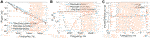FIGURE 2. Theoretical calculation and FEM simulation of the differential phase shifter with π phase difference. The orange shading marks the frequency range we choose to generate the π phase difference. (A) Theoretical and simulated phase response of the tube component (blue), theoretical phase response of the resonator component (black line), and simulated phase response of the resonator component (red line). (B) Theoretical and simulated amplitude responses of the tube and the resonator. (C) Theoretical and simulated phase difference between the two components.

The tube component and the resonator component in this π differential phase shifter will be used as the 0 and π phase modulators respectively to construct binary metasurfaces for reflected wave steering and focusing, which are two of the basic functions in wave control.

## 3 Binary Acoustic Reflection Metasurfaces Based on the Broadband π Differential Phase Shifter

### 3.1 Wave Steering

If we pattern the tubes (0 phase) and resonators (π phase) periodically, we get a binary phase grating for reflected acoustic waves. Binary phase grating is known to not have even order diffraction (O’Shea et al., 2004; Ma et al., 2019; Ma et al., 2020). By proper choice of the grating period, the energy in the reflected wave can be directed to one single direction. This design will lead to reflection metasurface realization for wave steering with simple structure and high efficiency.

In the coordinate system shown in Figure 3A, the pressure field $pin=ejkx0x$ incidents onto the binary phase grating. The angle of incidence, α, can be obtained from $sin(α)=kx0/k0$, where k0 = 2π/λ0 is the wavenumber in air, λ0 is the wavelength. The reflected wave Pr from a grating along the x direction at the plane y = 0 has the expression of $Pr=∑m=−∞∞Amej(kx0+mkg)x$, where kg = 2π/g is the grating constant, g is the grating period, and m is the diffraction order. The even diffraction order reflection A2N = 0, where N is a integer.

FIGURE 3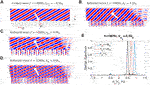FIGURE 3. Simulation of binary phase gratings for acoustic reflected wave steering. (A) Simulation setup and incident wave at $kx0=−0.5k0$(B–D) Reflected wave at different wave frequencies and grating constants: (B) f = 10 KHz and kg = 1.2k0, (C) f = 10 KHz and kg = 0.9k0, and (D) f = 15 KHz and kg = 0.9k0. (E) Spatial Fourier Transform of the reflected wave when the wave frequency is 10 KHz and the grating constant is kg = 1.4k0 (blue), kg = 1.2k0 (red), and kg = k0 (black).

Among the odd diffraction orders, when $|kx2N+1|, the (2N + 1)th diffraction order generates propagating wave. When $|kx2N+1|>k0$, the (2N + 1)th diffraction order generates evanescent wave that decays as it propagates away from the grating, thus carries no energy to the far-field. From $|kx2N+1|=|kx0+(2N+1)2πd|, we get the values of N that correspond to propagating wave components in the reflected wave. When the grating period g is small (which means the steering angle provided by the grating is large), all the diffraction orders except the zeroth order of the reflected wave are evanescent waves that do not carry any energy. All the wave energy are forced to the zeroth order. When d increases, the number of allowed N increases too. There is a range of d in which only one value of N is allowed. Further observation found that there are two cases when there is only one allowed N: N = −1 when $kx0>0$ and N = 0 when $kx0<0$, corresponding to the + 1st or − 1st order diffraction, respectively.

COMSOL simulation in frequency domain is performed for the designed gratings. In simulations, the gratings have a total length of 480 mm in the x direction. The grating is surrounded by perfect matching layers. A plane wave incidents from top of the grating, and the reflected wave distribution is calculated in COMSOL for different grating periods and different incident wave frequencies. When the incident wave has frequency f = 10 KHz and $kx0=−0.5k0$, the reflected field distributions are shown in Figures 3B,C for two different grating periods that give kg = 2π/g = 1.2k0 and 0.9k0. The incident wave is steered to different directions. We also plot the Spatial Fourier Transform of the reflected wave in the x direction for kg = 1.4k0, 1.2k0, and k0 in Figure 3E. The small peak at kx = −0.5k0 represents the 0th order diffraction in the reflected wave, and the peak at kx = 0.9k0, 0.7k0, and 0.5k0 are the first order diffractions in the reflected waves. We can see that the incident wave is steered to different directions with high efficiency by the binary phase grating with different grating periods.

Since the π phase difference between neighboring elements is preserved over frequency range from 10 KHz to 16 KHz in our phase shifter design, we choose two frequencies, 10 KHz and 15 KHz in this range, to demonstrate the unique behavior of the grating. Comparing 3) and 4) in Figure 3, the incident wave of 10 KHz and 15 KHz are steered to the same direction kx = 0.4k0 by the two binary phase gratings that have the same tube and resonator components but different grating periods.

Compared to the metasurfaces previously proposed, the binary phase grating has the advantage of simple structure with only two different phase modulation units while providing high steering efficiency. It also has the advantage of broadband phase shift values. Thus we no longer need to fabricate different phase shifters for different frequencies. We can fabricate the tubes and resonators as individual basic elements like Lego bricks, and then place the elements at different spatial locations for different working frequencies and/or steering angles.

### 3.2 Wave Focusing

As another example of the metasurfaces based on the broadband differential phase shifter, we demonstrate wave focusing function for reflected acoustic wave. In the coordinate system shown in Figure 4, the incident wave is a plane wave traveling in the -y direction. We design the sequence of the tube and resonator components in the metasurface that are aligned along the x direction in order to focus the reflected wave to a designated focal spot. The reflected waves at the locations of the tubes have the phase state of 0, and those at the locations of the resonators have the phase state of π. The binary phase patterns are designed with the angular momentum approach (Melde et al., 2016). In order to model the forward and backward acoustic wave propagation, the acoustic pressure at the focal plane (the plane in parallel with the x direction and at the y coordinate of the focal spot), pfocal, and right above the metasurface, pmetasurface will be decomposed into plane wave components with different spatial frequencies kxn:

$pmetasurface(x)=∑A(kxn)ej(kxnx)$
$pfocal(x)=∑B(kxn)ej(kxnx)$

where $B(kxn)=A(kxn)ejh0k02−kxn2$, and h0 is the projected distance in y direction between the metasurface and the focal spot. The binary phase patterns are calculated iteratively by forward and backward propagation of wave components with different spatial frequencies, as in Melde et al. (2016).

FIGURE 4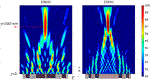FIGURE 4. Simulation of wave focusing at 10 KHz and 15 KHz. The incident wave is a plane wave travelling in the -y direction. The metasurfaces in both cases are formed by 35 equally spaced phase modulation elements and have the total size of 480 mm in the x direction. The sequence from the left to the right for the metasurface at 10 KHz is: (0,0,π, π, π,0, 0,0,0,0,π, π, π, π, π, π, π, 0, π, π, π, π, π, π, π, 0, 0, 0, 0, 0, π, π, π,0,0). That for the metasurface at 15 KHz is: (0,0,π, π, 0, 0, 0, π, π, π, 0, 0, 0, 0, 0, 0, 0, 0, 0, 0, 0, 0, 0, 0, 0, π, π, π, 0, 0, 0, π, π,0,0).

In the example shown in Figure 4, we design the metasurfaces to focus incident plane wave to a focal spot at h0 = 500 mm. Two working frequencies, 10 KHz and 15 kHz, are chosen to demonstrate the broadband behavior of the phase shifters in focusing metasurfaces. The size of both metasurfaces in x direction is 480 mm, with 35 phase modulation elements in total. The distance between neighboring elements is 13.7 mm, corresponding to half of the wavelength at frequency 12.5 KHz in air. The tubes and the resonators as in the differential π phase shifter are arranged in different sequences along x direction to form the metasurfaces for different frequencies. In both cases in Figure 4, the incident wave is focused to the focal spot with greater than 10 dB sound pressure level difference compared to other locations along the x direction when y = 500 mm. For each frequency in the range from 10 KHz to 16 kHz, which is the broadband working frequency range of the differential π phase shifter, we can achieve reflected wave focusing by arranging tubes and resonators into different sequences designed by the iterative design procedure without designing new phase shifters for each frequency.

## 4 Summary

In summary, we developed a method to design broadband differential phase shifters. Nearly constant phase difference between the two components in a phase shifter is generated over a range of frequencies. Using this method, we designed a broadband π differential phase shifter using a tube and a resonator. The nearly constant π phase difference between the tube and the resonator is preserved in frequency range from 10 KHz to 16 KHz. The tube and the resonator are further applied to the design of binary acoustic metasurfaces for reflected wave steering and focusing.

There are two main advantages of the metasurface design based on the broadband differential phase shifters. Firstly, we propose to achieve acoustic wave control using only two discrete phase shift values, 0 and π. Thus the metasurfaces we designed are much easier to fabricate and assemble, and the spatial phase variation frequency can be much larger than previous designs. Second, our phase shifter design is broadband. The phase shift values do not change with frequency. We can first fabricate the tubes and resonators as individual elements, and then tune the reflected wave angle (in wave steering function), focal location (in wave focusing function), and the working frequencies that those functions are achieved by simply changing the locations of those individual elements in the metasurface. We don’t need to re-fabricate the individual phase shifter elements for different frequencies.

The phase shifters can be fabricated with 3D printing techniques or template-based molding process. For applications in air, most of the solid materials can be used for the fabrication, such as metals, plastics, polymers, etc. The phase shifters serve as the basic building elements for metasurfaces that can be applied to in-door sound shaping, acoustic imaging, communication and energy harvesting applications.

## Data Availability Statement

The original contributions presented in the study are included in the article, further inquiries can be directed to the corresponding author.

## Author Contributions

CM conceived the presented idea. ZQ and XL performed the calculations and simulations. ZQ and CM drafted the manuscript. All authors discussed the results and contributed to the final manuscript.

## Conflict of Interest

The authors declare that the research was conducted in the absence of any commercial or financial relationships that could be construed as a potential conflict of interest.

## Publisher’s Note

All claims expressed in this article are solely those of the authors and do not necessarily represent those of their affiliated organizations, or those of the publisher, the editors and the reviewers. Any product that may be evaluated in this article, or claim that may be made by its manufacturer, is not guaranteed or endorsed by the publisher.

## Acknowledgments

CM acknowledges the funding support from Wisconsin Alumni Research Foundation and the University of Wisconsin Madison startup package.

## References

Beranek, L. L. (1954). Acoustics. New York: Acoust. Soc. Am).

Chen, H.-T., Taylor, A. J., and Yu, N. (2016). A Review of Metasurfaces: Physics and Applications. Rep. Prog. Phys. 79, 076401. doi:10.1088/0034-4885/79/7/076401

Cummer, S. A., Christensen, J., and Alù, A. (2016). Controlling Sound with Acoustic Metamaterials. Nat. Rev. Mater. 1, 16001. doi:10.1038/natrevmats.2016.1

Esfahlani, H., Karkar, S., Lissek, H., and Mosig, J. R. (2016). Acoustic Carpet Cloak Based on an Ultrathin Metasurface. Phys. Rev. B 94, 014302. doi:10.1103/physrevb.94.014302

Glybovski, S. B., Tretyakov, S. A., Belov, P. A., Kivshar, Y. S., and Simovski, C. R. (2016). Metasurfaces: From Microwaves to Visible. Phys. Rep. 634, 1–72. doi:10.1016/j.physrep.2016.04.004

Kildishev, A. V., Boltasseva, A., and Shalaev, V. M. (2013). Planar Photonics with Metasurfaces. Science 339, 1232009. doi:10.1126/science.1232009

Li, J., Shen, C., Díaz-Rubio, A., Tretyakov, S. A., and Cummer, S. A. (2018). Systematic Design and Experimental Demonstration of Bianisotropic Metasurfaces for Scattering-free Manipulation of Acoustic Wavefronts. Nat. Commun. 9, 1342. doi:10.1038/s41467-018-03778-9

Li, J., Wang, W., Xie, Y., Popa, B.-I., and Cummer, S. A. (2016). A Sound Absorbing Metasurface with Coupled Resonators. Appl. Phys. Lett. 109, 091908. doi:10.1063/1.4961671

Li, Y., Jiang, X., Li, R.-q., Liang, B., Zou, X.-y., Yin, L.-l., et al. (2014). Experimental Realization of Full Control of Reflected Waves with Subwavelength Acoustic Metasurfaces. Phys. Rev. Appl. 2, 064002. doi:10.1103/physrevapplied.2.064002

Li, Y., Jiang, X., Liang, B., Cheng, J.-c., and Zhang, L. (2015). Metascreen-based Acoustic Passive Phased Array. Phys. Rev. Appl. 4, 024003. doi:10.1103/physrevapplied.4.024003

Liang, Z., and Li, J. (2012). Extreme Acoustic Metamaterial by Coiling up Space. Phys. Rev. Lett. 108, 114301. doi:10.1103/physrevlett.108.114301

Ma, C., Kim, S., and Fang, N. X. (2019). Far-field Acoustic Subwavelength Imaging and Edge Detection Based on Spatial Filtering and Wave Vector Conversion. Nat. Commun. 10, 204. doi:10.1038/s41467-018-08221-7

Ma, C., Li, X., and Fang, N. X. (2020). Acoustic Angle-Selective Transmission Based on Binary Phase Gratings. Phys. Rev. Appl. 14, 064058. doi:10.1103/physrevapplied.14.064058

Ma, G., and Sheng, P. (2016). Acoustic Metamaterials: From Local Resonances to Broad Horizons. Sci. Adv. 2, e1501595. doi:10.1126/sciadv.1501595

Ma, G., Yang, M., Xiao, S., Yang, Z., and Sheng, P. (2014). Acoustic Metasurface with Hybrid Resonances. Nat. Mater 13, 873–878. doi:10.1038/nmat3994

Mei, J., Ma, G., Yang, M., Yang, Z., Wen, W., and Sheng, P. (2012). Dark Acoustic Metamaterials as Super Absorbers for Low-Frequency Sound. Nat. Commun. 3, 756. doi:10.1038/ncomms1758

Melde, K., Mark, A. G., Qiu, T., and Fischer, P. (2016). Holograms for Acoustics. Nature 537, 518–522. doi:10.1038/nature19755

O’Shea, D. C., Suleski, T. J., Kathman, A. D., and Prather, D. W. (2004). Diffractive Optics: Design, Fabrication, and Test, Vol. 62. (WA: Spie Press Bellingham).

Quirarte, J. L. R., and Starski, J. P. (1993). Novel Schiffman Phase Shifters. IEEE Trans. Microwave Theor. Techn. 41, 9–14. doi:10.1109/22.210223

Sun, S., He, Q., Xiao, S., Xu, Q., Li, X., and Zhou, L. (2012). Gradient-index Meta-Surfaces as a Bridge Linking Propagating Waves and Surface Waves. Nat. Mater 11, 426–431. doi:10.1038/nmat3292

Tang, K., Qiu, C., Ke, M., Lu, J., Ye, Y., and Liu, Z. (2014). Anomalous Refraction of Airborne Sound through Ultrathin Metasurfaces. Sci. Rep. 4, 6517. doi:10.1038/srep06517

Wang, W., Xie, Y., Popa, B.-I., and Cummer, S. A. (2016). Subwavelength Diffractive Acoustics and Wavefront Manipulation with a Reflective Acoustic Metasurface. J. Appl. Phys. 120, 195103. doi:10.1063/1.4967738

Xie, Y., Shen, C., Wang, W., Li, J., Suo, D., Popa, B.-I., et al. (2016). Acoustic Holographic Rendering with Two-Dimensional Metamaterial-Based Passive Phased Array. Sci. Rep. 6, 35437. doi:10.1038/srep35437

Xie, Y., Wang, W., Chen, H., Konneker, A., Popa, B.-I., and Cummer, S. A. (2014). Wavefront Modulation and Subwavelength Diffractive Acoustics with an Acoustic Metasurface. Nat. Commun. 5, 5553. doi:10.1038/ncomms6553

Yong-Xin Guo, Y.-X., Zhen-Yu Zhang, Z.-Y., and Ling Chuen Ong, L. C. (2006). Improved Wide-Band Schiffman Phase Shifter. IEEE Trans. Microwave Theor. Techn. 54, 1196–1200. doi:10.1109/tmtt.2005.864105

Yu, N., Genevet, P., Kats, M. A., Aieta, F., Tetienne, J.-P., Capasso, F., et al. (2011). Light Propagation with Phase Discontinuities: Generalized Laws of Reflection and Refraction. science 334, 333–337. doi:10.1126/science.1210713

Zhang, J., Lei Mei, Z., Ru Zhang, W., Yang, F., and Jun Cui, T. (2013). An Ultrathin Directional Carpet Cloak Based on Generalized Snell's Law. Appl. Phys. Lett. 103, 151115. doi:10.1063/1.4824898

Zhang, S., Yin, L., and Fang, N. (2009). Focusing Ultrasound with an Acoustic Metamaterial Network. Phys. Rev. Lett. 102, 194301. doi:10.1103/physrevlett.102.194301

Zhang, Z., Jiao, Y.-C., Cao, S.-F., Wang, X.-M., and Zhang, F.-S. (2011). Modified Broadband Schiffman Phase Shifter Using Dentate Microstrip and Patterned Ground Plane. PIER Lett. 24, 9–16. doi:10.2528/pierl11041406

Zhao, J., Li, B., Chen, Z., and Qiu, C.-W. (2013). Manipulating Acoustic Wavefront by Inhomogeneous Impedance and Steerable Extraordinary Reflection. Sci. Rep. 3, 2537. doi:10.1038/srep02537

Zheludev, N. I., and Kivshar, Y. S. (2012). From Metamaterials to Metadevices. Nat. Mater 11, 917–924. doi:10.1038/nmat3431

Zhu, X., Li, K., Zhang, P., Zhu, J., Zhang, J., Tian, C., et al. (2016). Implementation of Dispersion-free Slow Acoustic Wave Propagation and Phase Engineering with Helical-Structured Metamaterials. Nat. Commun. 7, 11731. doi:10.1038/ncomms11731

Zhu, Y., Hu, J., Fan, X., Yang, J., Liang, B., Zhu, X., et al. (2018). Fine Manipulation of Sound via Lossy Metamaterials with Independent and Arbitrary Reflection Amplitude and Phase. Nat. Commun. 9, 1632. doi:10.1038/s41467-018-04103-0

Keywords: focusing, steering, reflection, binary, broadband, metasurface, acoustics, differential phase shifter

Citation: Qin Z, Liu X and Ma C (2021) Acoustic Wave Reflection Control Based on Broadband Differential Phase Shifters. Front. Mech. Eng 7:703019. doi: 10.3389/fmech.2021.703019

Received: 30 April 2021; Accepted: 01 September 2021;
Published: 17 September 2021.

Edited by:

Philip Feng, University of Florida, United States

Reviewed by:

Yanan Wang, University of Nebraska-Lincoln, United States
Ruochen Lu, University of Texas at Austin, United States

Copyright © 2021 Qin, Liu and Ma. This is an open-access article distributed under the terms of the Creative Commons Attribution License (CC BY). The use, distribution or reproduction in other forums is permitted, provided the original author(s) and the copyright owner(s) are credited and that the original publication in this journal is cited, in accordance with accepted academic practice. No use, distribution or reproduction is permitted which does not comply with these terms.

*Correspondence: Chu Ma, chu.ma@wisc.edu

These authors have contributed equally to this work